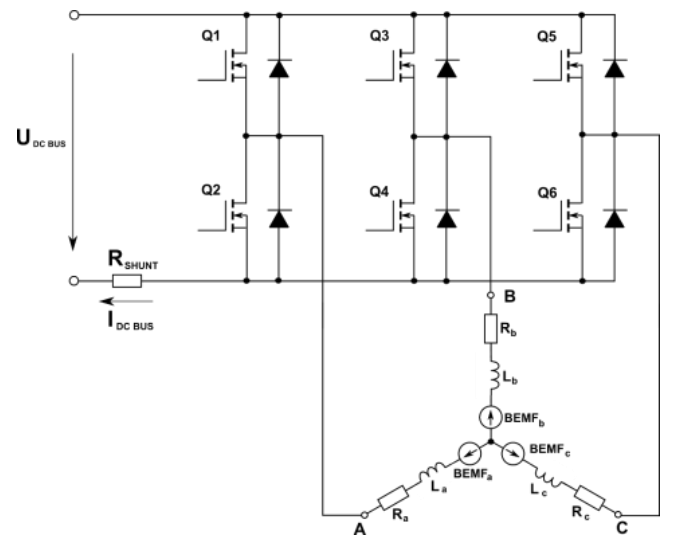## The decay period after commutating for BLDC

cancel
Showing results for
Did you mean:

## The decay period after commutating for BLDC

301 Views
Contributor III

During the motor rotation.In a sector, A is connected to the positive power source and B to the negative power source.After the following commutations (A connected to the positive, C connected to the negative), there was a decay period.Now, what is the current going to be flowing in?6 Replies
245 ViewsNXP TechSupport

Hi 相国 尹 ,

If my understanding of your question is correct:

The phase current can be measured reading the voltage across the R shunt resistor. However, this measurement cannot always be performed.  To get further details please refer to:

AN12435 3-Phase Sensorless BLDC Motor Control Kit with S32K144

section : 3.3.2. BEMF zero-crossing event detection and phase current measurement.

I apologize for my delayed reply and I hope this helps!

In the case you need further help, please let me know

Yours,

Charles

245 Views
Contributor III

Hi  Charles,

I mean, after commutation, what would the current loop look like during decay,

245 ViewsNXP TechSupport

Hi, XiangGuo,

Regarding your question, I think it is dependent the current flow direction. Based on your above figure,assume at a time, the Q1 and Q4 are ON, the current flows from A phase to B phase. For example , after the commutation, if the Q5 is ON, Q1 off, the desired current flows from Phase C to Phase B, and the Phase A is unpowered, in the case, Q2 diode will flow current and decays with time, the decay time is dependent on the motor phase resistor, inductor and the momentary current(load of the motor).  For example , after the commutation, if the Q6 is ON, Q4 off, the desired current flows from Phase A to Phase C, and the Phase B is unpowered, in the case, Q3 diode will flow current and charge the DC bus, decays with time.

BR

Xiangjun Rong

223 Views
Contributor III

1、Is the midpoint voltage of the three-phase winding constant?for UDCB/2?

2、After Q5 is on and Q1 is off, the B phase current is twice normal?

3、The current at Rshunt is bidirectional, right?

196 ViewsNXP TechSupport

Hi XiangGuo,

Here is the feedback that @xiangjun_rong kindly provide me:

Is the midpoint voltage of the three-phase winding constant?for UDCB/2?

You can check the AN12435  equation.

Where you can see the expression  for the voltage of the unpowered phase being equal to the  (UDBC/2) + (3/2*BEMF voltage of the unpowered phase)

2 After Q5 is on and Q1 is off, the B phase current is twice normal?

We do not think the current will be double in the branch switch, because the motor winding is an inductor, the current flowing an inductor can NOT increase immediately, it needs time.

The inductor current increment=  (V) *  (Ton / L)

Where: V is voltage to inductor, Ton is turning on time and  L is winding inductor.

3. The current at Rshunt is bidirectional, right?

Yes, the current at the Rshunt  is bi-directional.

But it is dependent on the layout and PWM waveform, based on the circuit that you provided us,

Lets take this scenario :

Assume that Q1/Q2 PWM signals are complementary, Q3/Q4 PWM signals are complementary,

Assume that at an instant, Q1/Q4 turn on, the current flows from DC bus to A winding via Q1, to B winding, to Q4, to GND to Rshunt.

At next instant, Q1/Q4 turn off, Q2/Q3 turn on, because of winding inductor, the current will keep from A winding to B Winding, this is the current flowing direction: A winding to B winding, to Q3 parallel diode, to DC bus(charging the power supply capacitor), to Rshunt, to Q2 parallel diode, to A winding.

So in ONE PWM cycle, the current flowing into Rshunt is in two directions.

I hope this helps !

Best regards,

Diego.

107 Views
Contributor III

1. It can be seen from AN12435 that uN = uDCB + eC / 2. So does the midpoint voltage change?

2. Assume at a time, the Q1 and Q4 are ON, the current flows from A phase to B phase. after the commutation, if Q5 conduction, Q1 is off.

Therefore, in the decay period, there is current in all three phases of A, B and C. What is the current direction on the DC bus?